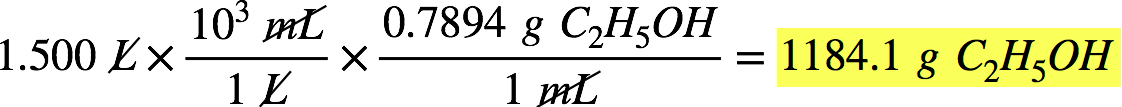# Problem: Outline the steps needed to solve the following problem, then do the calculations. Ether, (C2H5)2O, which was originally used as an anesthetic but has been replaced by safer and more effective medications, is prepared by the reaction of ethanol with sulfuric acid.2C2H5OH + H2SO4⟶ (C2H5)2O + H2SO4∙H2OWhat is the percent yield of ether if 1.17 L (d = 0.7134 g/mL) is isolated from the reaction of 1.500 L of C2H5OH (d = 0.7894 g/mL)?

🤓 Based on our data, we think this question is relevant for Professor Gunasekera's class at UIUC.

###### FREE Expert Solution

We first need to determine the theoretical yield of ether, (C2H5)2O, from the reaction. Recall that the theoretical yield is the expected amount of product formed in a reaction.

Using the given volume (1.500 L) and density (0.7894 g/mL) of C2H5OH, we can calculate the mass of C2H5OH consumed in the reaction:###### Problem Details

Outline the steps needed to solve the following problem, then do the calculations. Ether, (C2H5)2O, which was originally used as an anesthetic but has been replaced by safer and more effective medications, is prepared by the reaction of ethanol with sulfuric acid.

2C2H5OH + H2SO4⟶ (C2H5)2O + H2SO4∙H2O

What is the percent yield of ether if 1.17 L (d = 0.7134 g/mL) is isolated from the reaction of 1.500 L of C2H5OH (d = 0.7894 g/mL)?